# R S Aggarwal Solutions for Class 10 Maths Chapter 5 Arithmetic Progression Exercise 5D

R S Aggarwal Solutions for Class 10 Maths exercise 5d are available here. Students can get detailed step-by-step explanations to all the questions listed under exercise 5d of Arithmetic Progression of the Class 10 R S Aggarwal Textbook. Download pdf of Class 10 Maths Chapter 5 R S Aggarwal Solutions and accelerate your knowledge.

## Download PDF of R S Aggarwal Solutions for Class 10 Maths Chapter 5 Arithmetic Progression Exercise 5D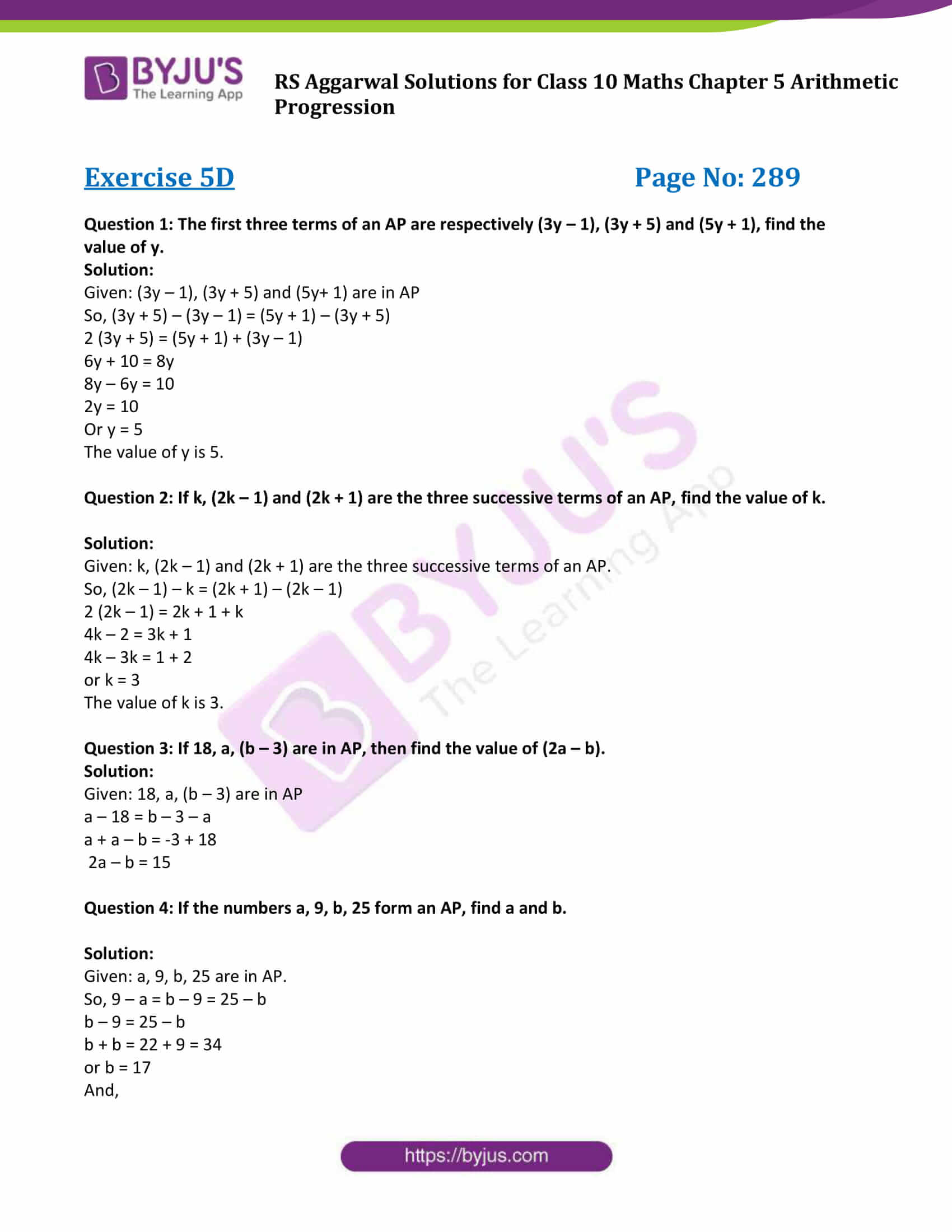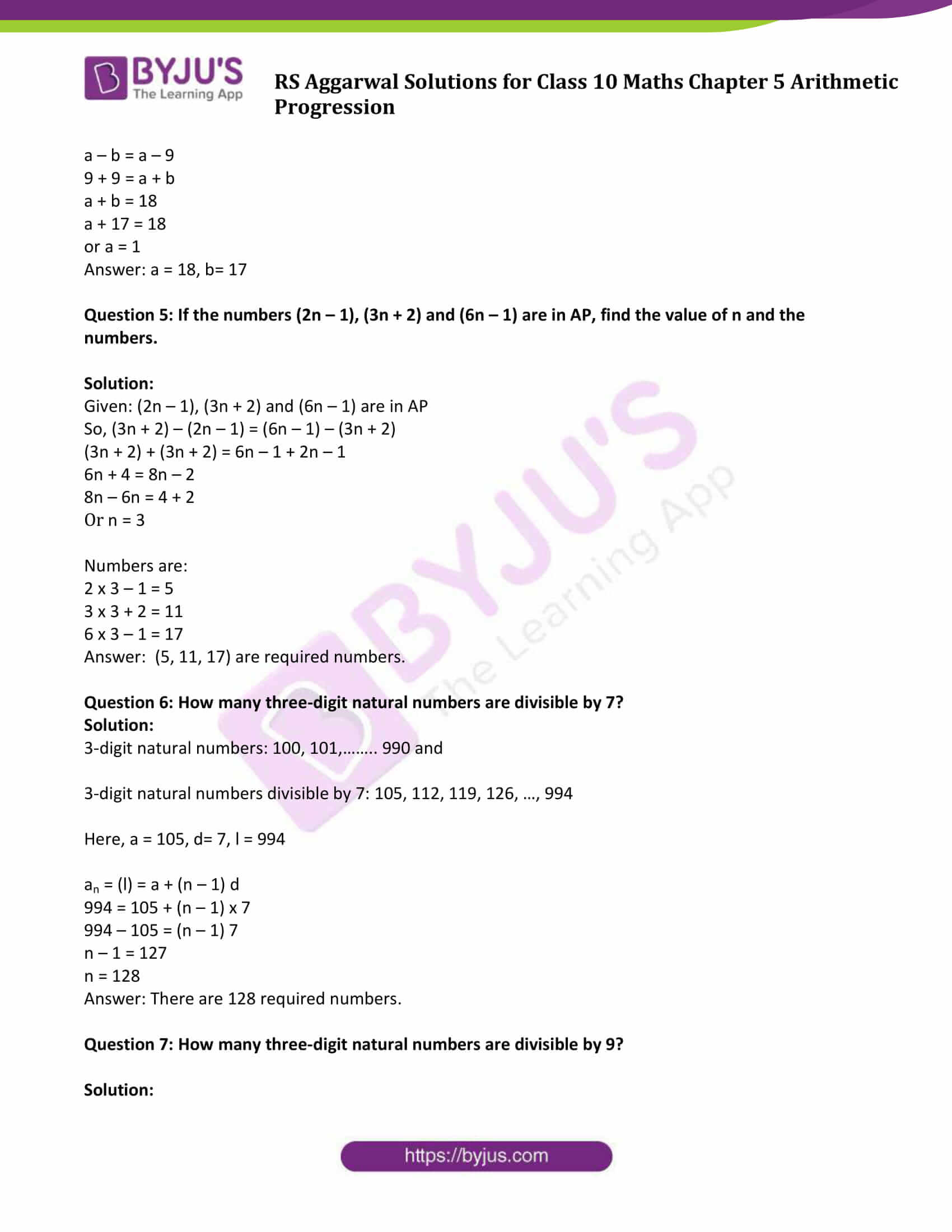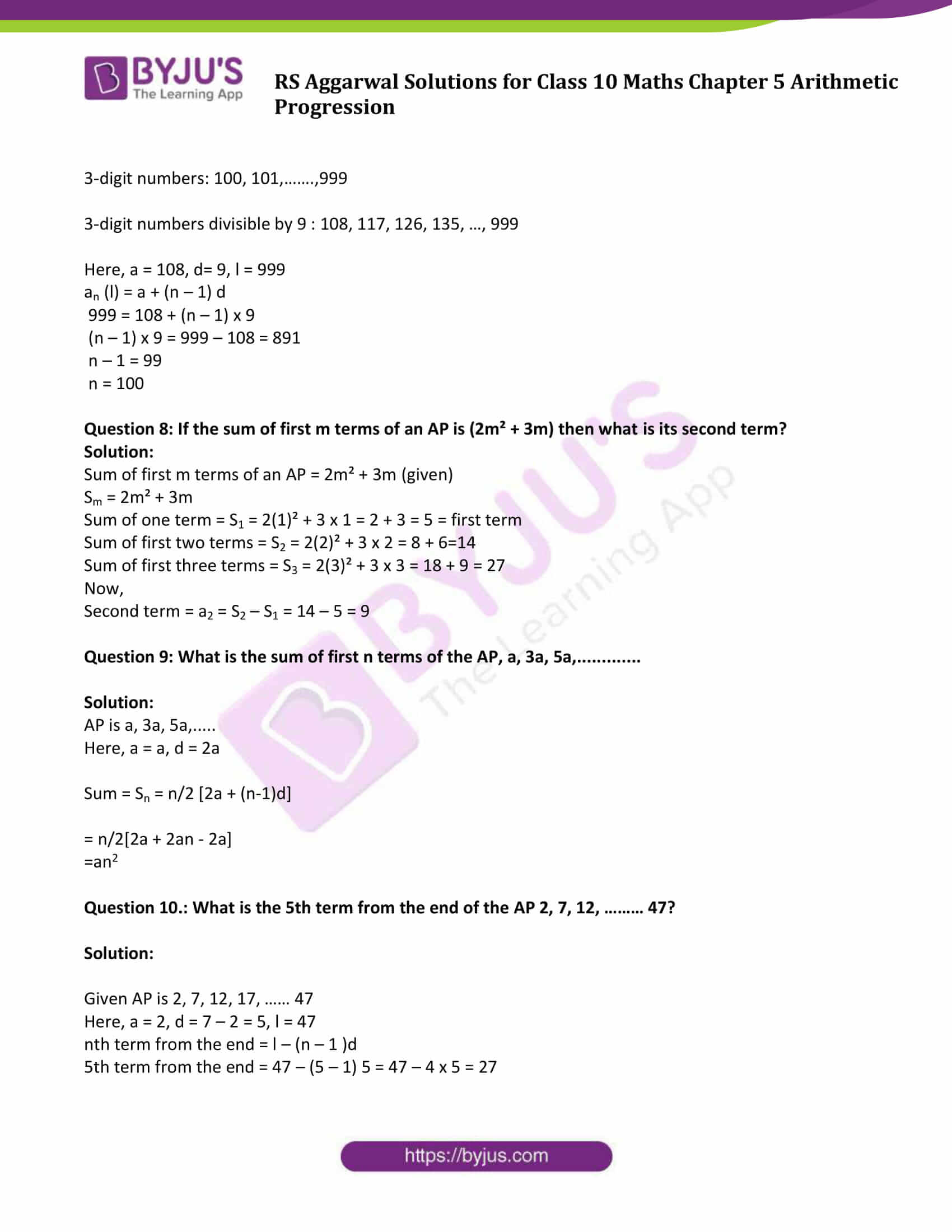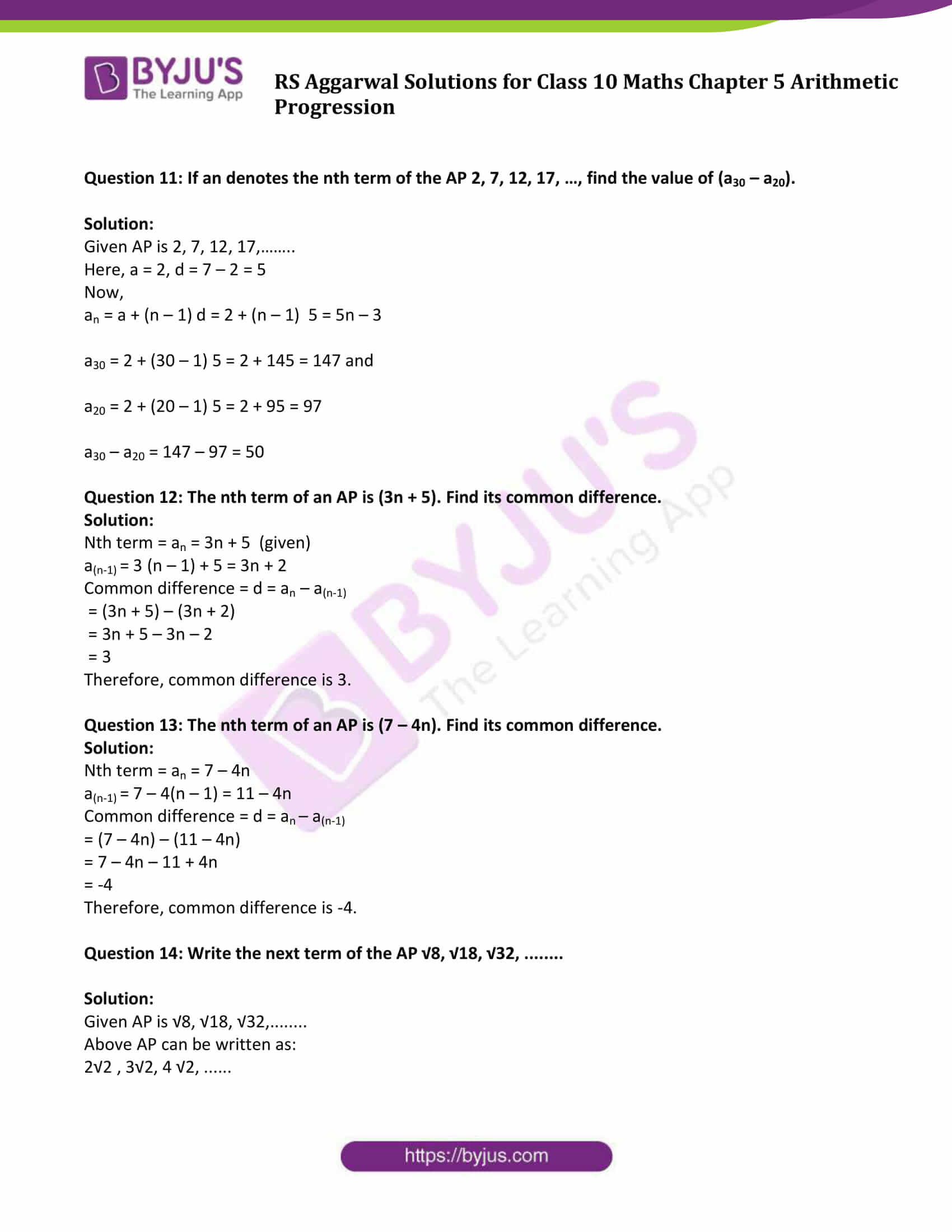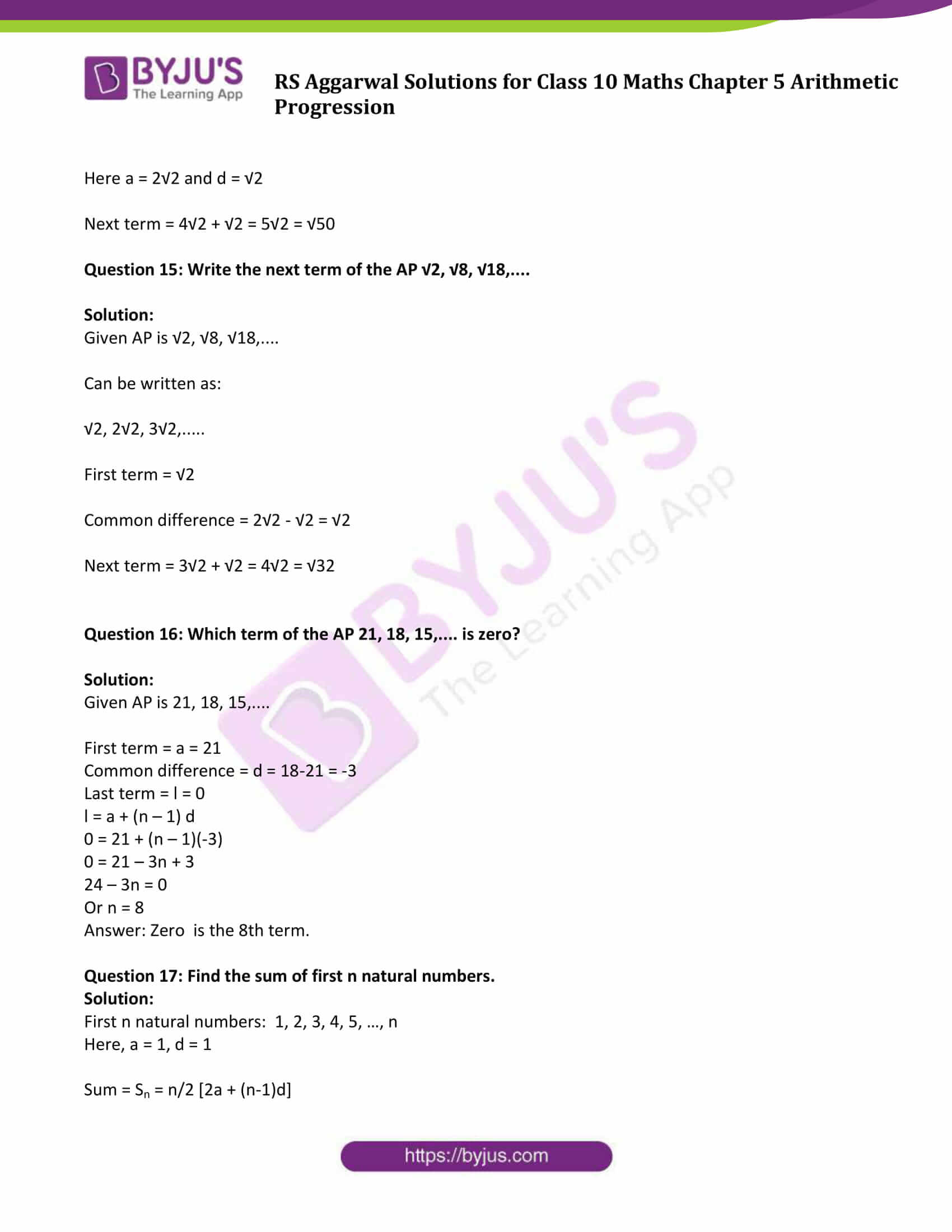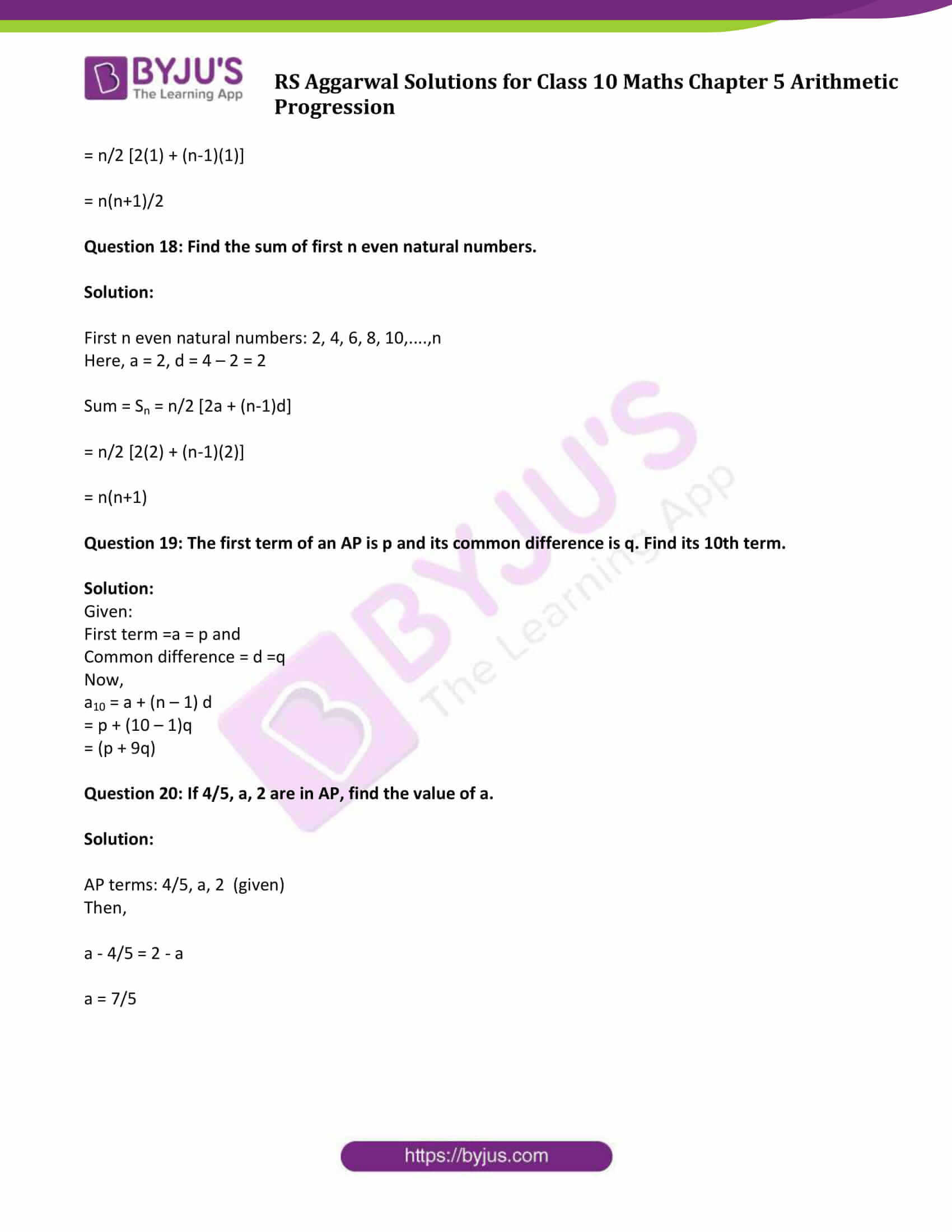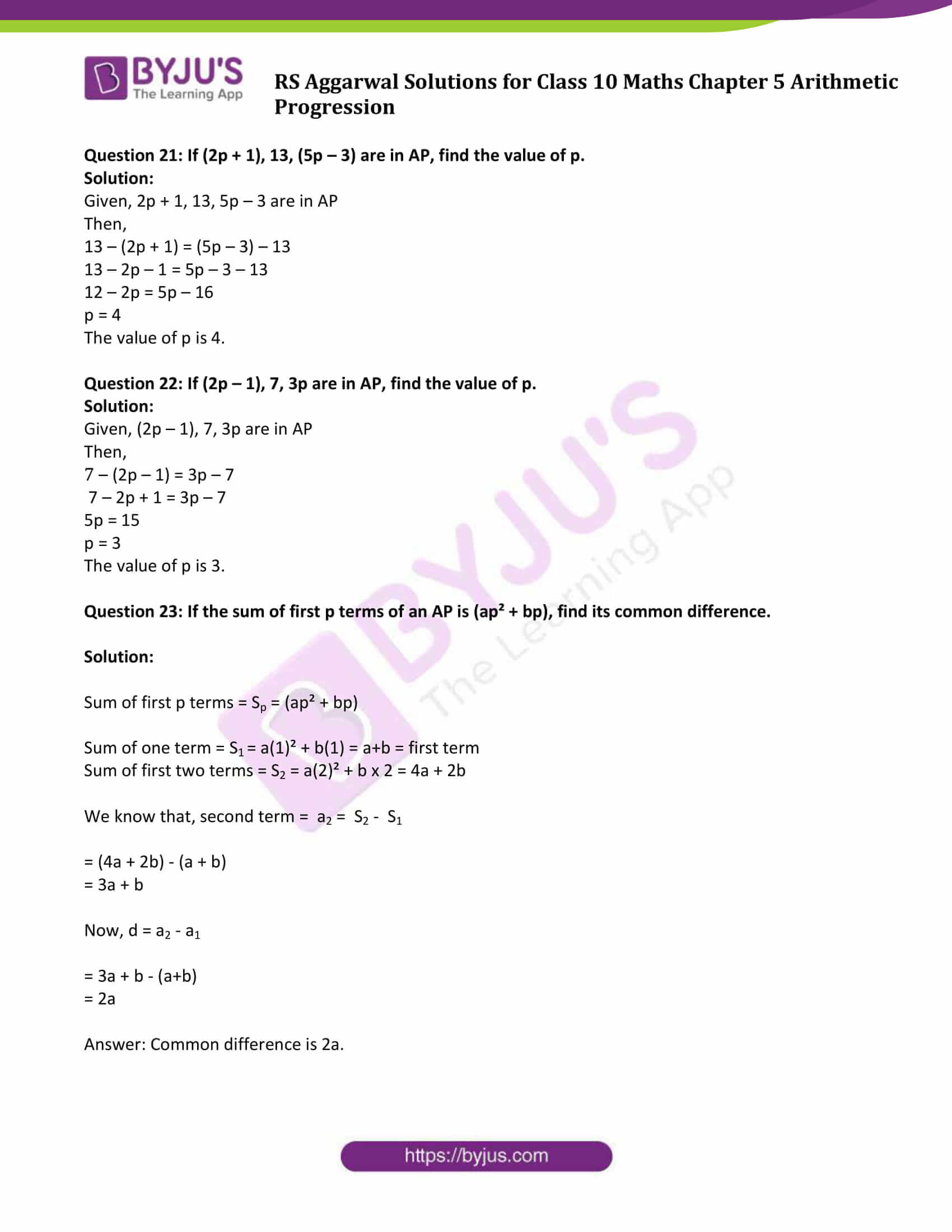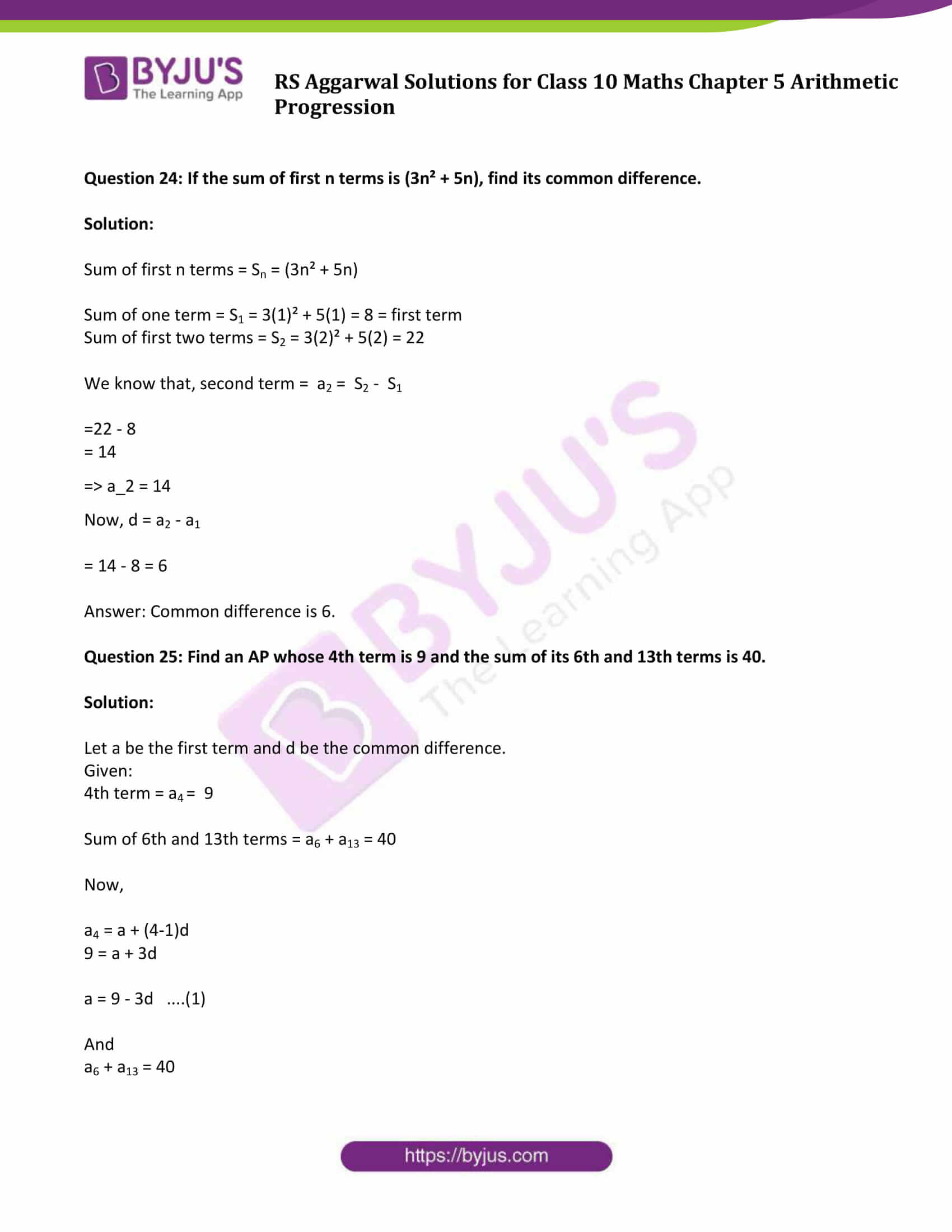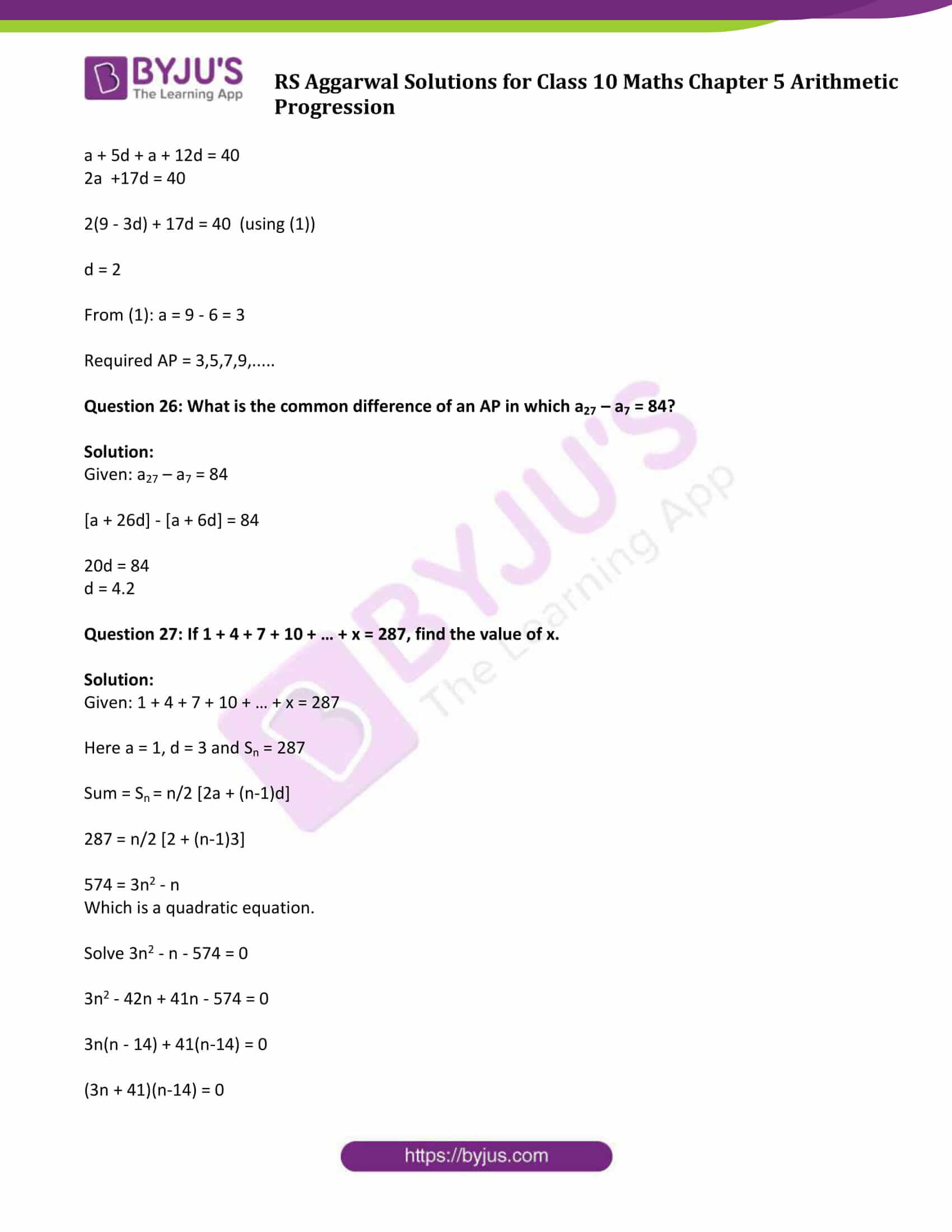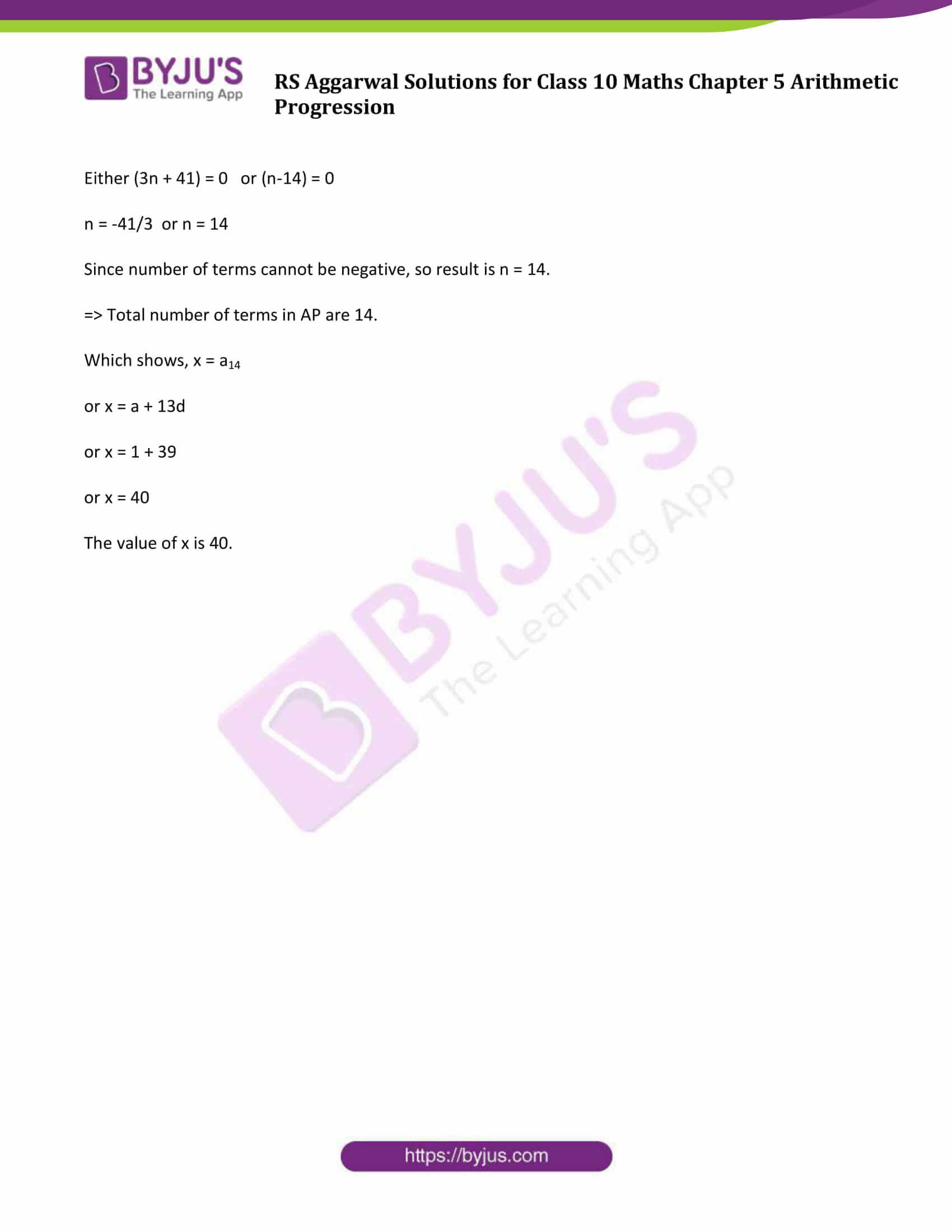## Access other exercise solutions of Class 10 Maths Chapter 5 Arithmetic Progression

Exercise 5A Solutions: 30 Questions (Short Answers)

Exercise 5B Solutions: 16 Questions (Short Answers)

Exercise 5C Solutions: 15 Questions (Short Answers)

## Exercise 5D Page No: 289

Question 1: The first three terms of an AP are respectively (3y – 1), (3y + 5) and (5y + 1), find the value of y.

Solution:

Given: (3y – 1), (3y + 5) and (5y+ 1) are in AP

So, (3y + 5) – (3y – 1) = (5y + 1) – (3y + 5)

2 (3y + 5) = (5y + 1) + (3y – 1)

6y + 10 = 8y

8y – 6y = 10

2y = 10

Or y = 5

The value of y is 5.

Question 2: If k, (2k – 1) and (2k + 1) are the three successive terms of an AP, find the value of k.

Solution:

Given: k, (2k – 1) and (2k + 1) are the three successive terms of an AP.

So, (2k – 1) – k = (2k + 1) – (2k – 1)

2 (2k – 1) = 2k + 1 + k

4k – 2 = 3k + 1

4k – 3k = 1 + 2

or k = 3

The value of k is 3.

Question 3: If 18, a, (b – 3) are in AP, then find the value of (2a – b).

Solution:

Given: 18, a, (b – 3) are in AP

a – 18 = b – 3 – a

a + a – b = -3 + 18

2a – b = 15

Question 4: If the numbers a, 9, b, 25 form an AP, find a and b.

Solution:

Given: a, 9, b, 25 are in AP.

So, 9 – a = b – 9 = 25 – b

b – 9 = 25 – b

b + b = 22 + 9 = 34

or b = 17

And,

a – b = a – 9

9 + 9 = a + b

a + b = 18

a + 17 = 18

or a = 1

Answer: a = 18, b= 17

Question 5: If the numbers (2n – 1), (3n + 2) and (6n – 1) are in AP, find the value of n and the numbers.

Solution:

Given: (2n – 1), (3n + 2) and (6n – 1) are in AP

So, (3n + 2) – (2n – 1) = (6n – 1) – (3n + 2)

(3n + 2) + (3n + 2) = 6n – 1 + 2n – 1

6n + 4 = 8n – 2

8n – 6n = 4 + 2

Or n = 3

Numbers are:

2 x 3 – 1 = 5

3 x 3 + 2 = 11

6 x 3 – 1 = 17

Answer: (5, 11, 17) are required numbers.

Question 6: How many three-digit natural numbers are divisible by 7?

Solution:

3-digit natural numbers: 100, 101,…….. 990 and

3-digit natural numbers divisible by 7: 105, 112, 119, 126, …, 994

Here, a = 105, d= 7, l = 994

an = (l) = a + (n – 1) d

994 = 105 + (n – 1) x 7

994 – 105 = (n – 1) 7

n – 1 = 127

n = 128

Answer: There are 128 required numbers.

Question 7: How many three-digit natural numbers are divisible by 9?

Solution:

3-digit numbers: 100, 101,…….,999

3-digit numbers divisible by 9 : 108, 117, 126, 135, …, 999

Here, a = 108, d= 9, l = 999

an (l) = a + (n – 1) d

999 = 108 + (n – 1) x 9

(n – 1) x 9 = 999 – 108 = 891

n – 1 = 99

n = 100

Question 8: If the sum of first m terms of an AP is (2m² + 3m) then what is its second term?

Solution:

Sum of first m terms of an AP = 2m² + 3m (given)

Sm = 2m² + 3m

Sum of one term = S1 = 2(1)² + 3 x 1 = 2 + 3 = 5 = first term

Sum of first two terms = S2 = 2(2)² + 3 x 2 = 8 + 6=14

Sum of first three terms = S3 = 2(3)² + 3 x 3 = 18 + 9 = 27

Now,

Second term = a2 = S2 – S1 = 14 – 5 = 9

Question 9: What is the sum of first n terms of the AP, a, 3a, 5a,………….

Solution:

AP is a, 3a, 5a,…..

Here, a = a, d = 2a

Sum = Sn = n/2 [2a + (n-1)d]

= n/2[2a + 2an – 2a]

=an2

Question 10.: What is the 5th term from the end of the AP 2, 7, 12, ……… 47?

Solution:

Given AP is 2, 7, 12, 17, …… 47

Here, a = 2, d = 7 – 2 = 5, l = 47

nth term from the end = l – (n – 1 )d

5th term from the end = 47 – (5 – 1) 5 = 47 – 4 x 5 = 27

Question 11: If an denotes the nth term of the AP 2, 7, 12, 17, …, find the value of (a30 – a20).

Solution:

Given AP is 2, 7, 12, 17,……..

Here, a = 2, d = 7 – 2 = 5

Now,

an = a + (n – 1) d = 2 + (n – 1) 5 = 5n – 3

a30 = 2 + (30 – 1) 5 = 2 + 145 = 147 and

a20 = 2 + (20 – 1) 5 = 2 + 95 = 97

a30 – a20 = 147 – 97 = 50

Question 12: The nth term of an AP is (3n + 5). Find its common difference.

Solution:

Nth term = an = 3n + 5 (given)

a(n-1) = 3 (n – 1) + 5 = 3n + 2

Common difference = d = an – a(n-1)

= (3n + 5) – (3n + 2)

= 3n + 5 – 3n – 2

= 3

Therefore, common difference is 3.

Question 13: The nth term of an AP is (7 – 4n). Find its common difference.

Solution:

Nth term = an = 7 – 4n

a(n-1) = 7 – 4(n – 1) = 11 – 4n

Common difference = d = an – a(n-1)

= (7 – 4n) – (11 – 4n)

= 7 – 4n – 11 + 4n

= -4

Therefore, common difference is -4.

Question 14: Write the next term of the AP √8, √18, √32, ……..

Solution:

Given AP is √8, √18, √32,……..

Above AP can be written as:

2√2 , 3√2, 4 √2, ……

Here a = 2√2 and d = √2

Next term = 4√2 + √2 = 5√2 = √50

Question 15: Write the next term of the AP √2, √8, √18,….

Solution:

Given AP is √2, √8, √18,….

Can be written as:

√2, 2√2, 3√2,…..

First term = √2

Common difference = 2√2 – √2 = √2

Next term = 3√2 + √2 = 4√2 = √32

Question 16: Which term of the AP 21, 18, 15,…. is zero?

Solution:

Given AP is 21, 18, 15,….

First term = a = 21

Common difference = d = 18-21 = -3

Last term = l = 0

l = a + (n – 1) d

0 = 21 + (n – 1)(-3)

0 = 21 – 3n + 3

24 – 3n = 0

Or n = 8

Answer: Zero is the 8th term.

Question 17: Find the sum of first n natural numbers.

Solution:

First n natural numbers: 1, 2, 3, 4, 5, …, n

Here, a = 1, d = 1

Sum = Sn = n/2 [2a + (n-1)d]

= n/2 [2(1) + (n-1)(1)]

= n(n+1)/2

Question 18: Find the sum of first n even natural numbers.

Solution:

First n even natural numbers: 2, 4, 6, 8, 10,….,n

Here, a = 2, d = 4 – 2 = 2

Sum = Sn = n/2 [2a + (n-1)d]

= n/2 [2(2) + (n-1)(2)]

= n(n+1)

Question 19: The first term of an AP is p and its common difference is q. Find its 10th term.

Solution:

Given:

First term =a = p and

Common difference = d =q

Now,

a10 = a + (n – 1) d

= p + (10 – 1)q

= (p + 9q)

Question 20: If 4/5, a, 2 are in AP, find the value of a.

Solution:

AP terms: 4/5, a, 2 (given)

Then,

a – 4/5 = 2 – a

a = 7/5

Question 21: If (2p + 1), 13, (5p – 3) are in AP, find the value of p.

Solution:

Given, 2p + 1, 13, 5p – 3 are in AP

Then,

13 – (2p + 1) = (5p – 3) – 13

13 – 2p – 1 = 5p – 3 – 13

12 – 2p = 5p – 16

p = 4

The value of p is 4.

Question 22: If (2p – 1), 7, 3p are in AP, find the value of p.

Solution:

Given, (2p – 1), 7, 3p are in AP

Then,

7 – (2p – 1) = 3p – 7

7 – 2p + 1 = 3p – 7

5p = 15

p = 3

The value of p is 3.

Question 23: If the sum of first p terms of an AP is (ap² + bp), find its common difference.

Solution:

Sum of first p terms = Sp = (ap² + bp)

Sum of one term = S1 = a(1)² + b(1) = a+b = first term

Sum of first two terms = S2 = a(2)² + b x 2 = 4a + 2b

We know that, second term = a2 = S2 – S1

= (4a + 2b) – (a + b)

= 3a + b

Now, d = a2 – a1

= 3a + b – (a+b)

= 2a

Question 24: If the sum of first n terms is (3n² + 5n), find its common difference.

Solution:

Sum of first n terms = Sn = (3n² + 5n)

Sum of one term = S1 = 3(1)² + 5(1) = 8 = first term

Sum of first two terms = S2 = 3(2)² + 5(2) = 22

We know that, second term = a2 = S2 – S1

=22 – 8

= 14

=> a2 = 14

Now, d = a2 – a1

= 14 – 8 = 6

Question 25: Find an AP whose 4th term is 9 and the sum of its 6th and 13th terms is 40.

Solution:

Let a be the first term and d be the common difference.

Given:

4th term = a4 = 9

Sum of 6th and 13th terms = a6 + a13 = 40

Now,

a4 = a + (4-1)d

9 = a + 3d

a = 9 – 3d ….(1)

And

a6 + a13 = 40

a + 5d + a + 12d = 40

2a +17d = 40

2(9 – 3d) + 17d = 40 (using (1))

d = 2

From (1): a = 9 – 6 = 3

Required AP = 3,5,7,9,…..

Question 26: What is the common difference of an AP in which a27 – a7 = 84?

Solution:

Given: a27 – a7 = 84

[a + 26d] – [a + 6d] = 84

20d = 84

d = 4.2

Question 27: If 1 + 4 + 7 + 10 + … + x = 287, find the value of x.

Solution:

Given: 1 + 4 + 7 + 10 + … + x = 287

Here a = 1, d = 3 and Sn = 287

Sum = Sn = n/2 [2a + (n-1)d]

287 = n/2 [2 + (n-1)3]

574 = 3n2 – n

Solve 3n2 – n – 574 = 0

3n2 – 42n + 41n – 574 = 0

3n(n – 14) + 41(n-14) = 0

(3n + 41)(n-14) = 0

Either (3n + 41) = 0 or (n-14) = 0

n = -41/3 or n = 14

Since number of terms cannot be negative, so result is n = 14.

=> Total number of terms in AP are 14.

Which shows, x = a14

or x = a + 13d

or x = 1 + 39

or x = 40

The value of x is 40.

## R S Aggarwal Solutions for Class 10 Maths Chapter 5 Arithmetic Progression Exercise 5D

Class 10 Maths Chapter 5 Arithmetic Progression Exercise 5D is based on the following topics:

• General term of an AP: an = a + (n-1)d
• nth term from the end of an AP
• Arithmetic mean between two numbers
• Sum of n terms of an AP